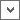# Define a Calculate Column for a Matrix Widget

Use the Columns page (in the Matrix Widget Properties window) to define how a calculated column looks and behaves on a Matrix Widget.

A calculated column displays a calculated value that is determined by executing a calculation against other columns in the Matrix (example: Add up open and closed Incidents for a grand total).

The following figure shows an example of how a Matrix Widget uses a calculated column on a Dashboard.Good to know:

• Calculated columns are calculated after all numeric columns have been retrieved.
• Calculated columns cannot query themselves.

To define a calculated column for a Matrix Widget:

1. Create a Widget.
2. In the Type drop-down, select Matrix.
3. Click the Columns page.
2. Click the Selector button, and then select the Tokens (System Functions, Expressions, Stored Values, Metrics, and/or Counters) and math operators to build the calculation.